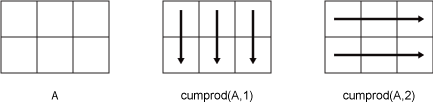# cumprod

Symbolic cumulative product

## Syntax

``B = cumprod(A)``
``B = cumprod(A,dim)``
``B = cumprod(___,direction)``

## Description

example

````B = cumprod(A)` returns an array the same size as `A` containing the cumulative product.If `A` is a vector, then `cumprod(A)` returns a vector containing the cumulative product of the elements of `A`.If `A` is a matrix, then `cumprod(A)` returns a matrix containing the cumulative products of each column of `A`.```

example

````B = cumprod(A,dim)` returns the cumulative product along dimension `dim`. For example, if `A` is a matrix, then `cumprod(A,2)` returns the cumulative product of each row.```

example

````B = cumprod(___,direction)` specifies the direction using any of the previous syntaxes. For instance, `cumprod(A,2,'reverse')` returns the cumulative product within the rows of `A` by working from end to beginning of the second dimension.```

## Examples

### Cumulative Product of Vector

Create a vector and find the cumulative product of its elements.

```V = 1./factorial(sym([1:5])) prod_V = cumprod(V)```
```V = [ 1, 1/2, 1/6, 1/24, 1/120] prod_V = [ 1, 1/2, 1/12, 1/288, 1/34560]```

### Cumulative Product of Each Column in Symbolic Matrix

Create matrix a 4-by-4 symbolic matrix `X` all elements of which equal `x`.

```syms x X = x*ones(4,4)```
```X = [ x, x, x, x] [ x, x, x, x] [ x, x, x, x] [ x, x, x, x]```

Compute the cumulative product of the elements of `X`. By default, `cumprod` returns the cumulative product of each column.

`productX = cumprod(X)`
```productX = [ x, x, x, x] [ x^2, x^2, x^2, x^2] [ x^3, x^3, x^3, x^3] [ x^4, x^4, x^4, x^4]```

### Cumulative Product of Each Row in Symbolic Matrix

Create matrix a 4-by-4 symbolic matrix, all elements of which equal `x`.

```syms x X = x*ones(4,4)```
```X = [ x, x, x, x] [ x, x, x, x] [ x, x, x, x] [ x, x, x, x]```

Compute the cumulative product of each row of the matrix `X`.

`productX = cumprod(X,2)`
```productX = [ x, x^2, x^3, x^4] [ x, x^2, x^3, x^4] [ x, x^2, x^3, x^4] [ x, x^2, x^3, x^4]```

### Reverse Cumulative Product

Create matrix a 4-by-4 symbolic matrix `X` all elements of which equal `x`.

```syms x X = x*ones(4,4)```
```X = [ x, x, x, x] [ x, x, x, x] [ x, x, x, x] [ x, x, x, x]```

Calculate the cumulative product along the columns in both directions. Specify the `'reverse'` option to work from right to left in each row.

```columnsDirect = cumprod(X) columnsReverse = cumprod(X,'reverse')```
```columnsDirect = [ x, x, x, x] [ x^2, x^2, x^2, x^2] [ x^3, x^3, x^3, x^3] [ x^4, x^4, x^4, x^4] columnsReverse = [ x^4, x^4, x^4, x^4] [ x^3, x^3, x^3, x^3] [ x^2, x^2, x^2, x^2] [ x, x, x, x]```

Calculate the cumulative product along the rows in both directions. Specify the `'reverse'` option to work from right to left in each row.

```rowsDirect = cumprod(X,2) rowsReverse = cumprod(X,2,'reverse')```
```rowsDirect = [ x, x^2, x^3, x^4] [ x, x^2, x^3, x^4] [ x, x^2, x^3, x^4] [ x, x^2, x^3, x^4] rowsReverse = [ x^4, x^3, x^2, x] [ x^4, x^3, x^2, x] [ x^4, x^3, x^2, x] [ x^4, x^3, x^2, x]```

## Input Arguments

collapse all

Input array, specified as a vector or matrix.

Dimension to operate along, specified as a positive integer. The default value is 1.

Consider a two-dimensional input array, `A`.

• `cumprod(A,1)` ) works on successive elements in the columns of `A` and returns the cumulative product of each column.

• `cumprod(A,2)` works on successive elements in the rows of `A` and returns the cumulative product of each row.`cumprod` returns `A` if `dim` is greater than `ndims(A)`.

Direction of cumulation, specified as the `'forward'` (default) or `'reverse'`.

• `'forward'` works from `1` to `end` of the active dimension.

• `'reverse'` works from `end` to `1` of the active dimension.

## Output Arguments

collapse all

Cumulative product array, returned as a vector or matrix of the same size as the input `A`.